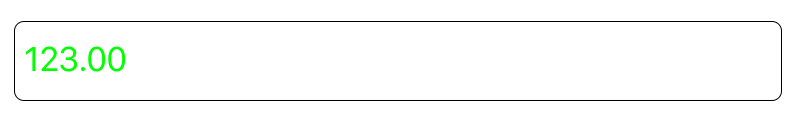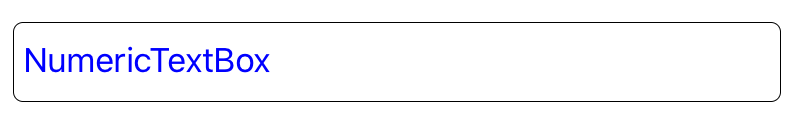# Color Customization in SfNumericTextBox

17 Jul 20201 minute to read

SfNumericTextBox is used to set custom background, text, and border colors through the following properties:

• `TextColor` - Sets the color of numeric textBox’s value

• `BackgroundColor` - Sets the background color of numeric textBox.

• `BorderColor` - Sets the border custom color of numeric textBox

• `WatermarkColor` - Sets the watermark custom color of numeric textBox’s watermark Text.

## TextColor

The following code sample demonstrates how to set the TextColor:

• C#
• ``````[C#]

SfNumericTextBox numericTextBox = new SfNumericTextBox()
{
TextColor = Color.Green,
Value = 123
};``````## BackgroundColor

The following code sample demonstrates how to set the BackgroundColor:

• C#
• ``````[C#]

SfNumericTextBox numericTextBox = new SfNumericTextBox()
{
BackgroundColor = Color.Red,
TextColor = Color.White
};``````## BorderColor

The following code sample demonstrates how to set the BorderColor color:

• C#
• ``````[C#]

SfNumericTextBox numericTextBox = new SfNumericTextBox()
{
BorderColor = UIColor.Blue
};``````## WatermarkColor

The following code sample demonstrates how to set the WatermarkColor:

• C#
• ``````[C#]

SfNumericTextBox numericTextBox = new SfNumericTextBox()
{
Watermark = (NSString)"NumericTextBox",
WatermarkColor = UIColor.Blue,
AllowNull=true
};``````